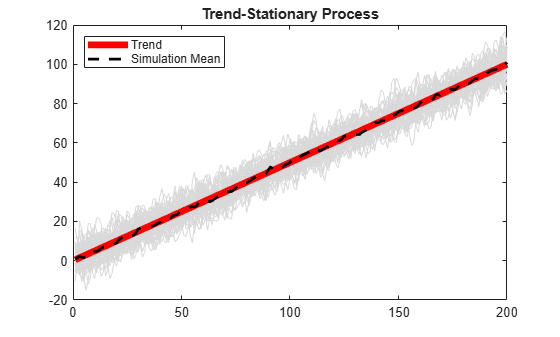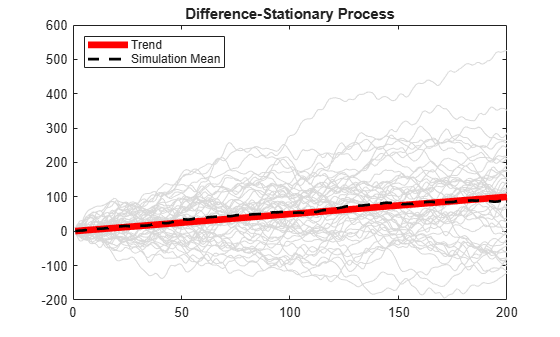Simulate Trend-Stationary and Difference-Stationary Processes

This example shows how to simulate trend-stationary and difference-stationary processes. The simulation results illustrate the distinction between these two nonstationary process models.

Generate Observations from Trend-Stationary Process

Specify the trend-stationary process

${y}_{t}=0.5t+{\epsilon }_{t}+1.4{\epsilon }_{t-1}+0.8{\epsilon }_{t-2},$

where the innovation process is Gaussian with variance 8. After specifying the model, simulate 50 sample paths of length 200. Use 100 burn-in simulations.

t = [1:200]';
trend = 0.5*t;

MdlTS = arima('Constant',0,'MA',{1.4,0.8},'Variance',8);
rng('default')
u = simulate(MdlTS,300,'NumPaths',50);

Yt = repmat(trend,1,50) + u(101:300,:);

figure
plot(Yt,'Color',[.85,.85,.85])
hold on
h1 = plot(t,trend,'r','LineWidth',5);
xlim([0,200])
title('Trend-Stationary Process')
h2 = plot(mean(Yt,2),'k--','LineWidth',2);
legend([h1,h2],'Trend','Simulation Mean',...
'Location','NorthWest')
hold offThe sample paths fluctuate around the theoretical trend line with constant variance. The simulation mean is close to the true trend line.

Generate Observations from Difference-Stationary Process

Specify the difference-stationary model

$\Delta {y}_{t}=0.5+{\epsilon }_{t}+1.4{\epsilon }_{t-1}+0.8{\epsilon }_{t-1},$

where the innovation distribution is Gaussian with variance 8. After specifying the model, simulate 50 sample paths of length 200. No burn-in is needed because all sample paths should begin at zero. This is the simulate default starting point for nonstationary processes with no presample data.

MdlDS = arima('Constant',0.5,'D',1,'MA',{1.4,0.8},...
'Variance',8);
Yd = simulate(MdlDS,200,'NumPaths',50);
figure
plot(Yd,'Color',[.85,.85,.85])
hold on
h1=plot(t,trend,'r','LineWidth',5);
xlim([0,200])
title('Difference-Stationary Process')
h2=plot(mean(Yd,2),'k--','LineWidth',2);
legend([h1,h2],'Trend','Simulation Mean',...
'Location','NorthWest')
hold offThe simulation average is close to the trend line with slope 0.5. The variance of the sample paths grows over time.

Difference Sample Paths

A difference-stationary process is stationary when differenced appropriately. Take the first differences of the sample paths from the difference-stationary process, and plot the differenced series. One observation is lost as a result of the differencing.

diffY = diff(Yd,1,1);

figure
plot(2:200,diffY,'Color',[.85,.85,.85])
xlim([0,200])
title('Differenced Series')
hold on
h = plot(2:200,mean(diffY,2),'k--','LineWidth',2);
legend(h,'Simulation Mean','Location','NorthWest')
hold offThe differenced series appears stationary, with the simulation mean fluctuating around zero.